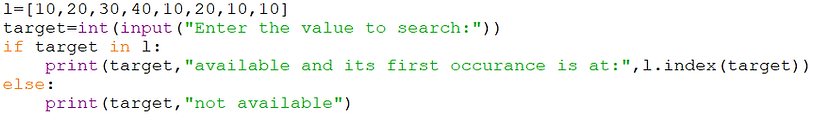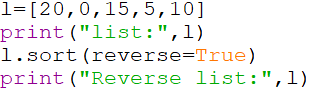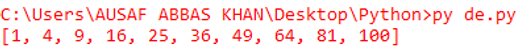top of page## Python: Working with List

List

If you want to represent a group of individual object as a single entity then list should be used.

In list:

• Insertion order is preserved.

• Duplicates are allowed.

• Heterogeneous objects are  allowed.

• List is growable in nature means based on our requirement we can increase or decrease size of a list.

How to create list object

• l = [] to create an empty list

• l = [10,20,30,40,50] if you already know value

• l = eval(input(‘Enter some list’)) if we want values dynamically from keyboard.

• l = list(sequence) by using list function

• l = s.split(separator) string to list conversion

Nested List

A list can contain another list, such type of list is called nested list.

Example: [10,20,[30,40]]

Accessing list elements

1. By using index (forward and backward direction).

2. By using slice operator.

Example:

l=[]

Type(l)------>list

l.append(10)

l.append(20)

l.append(30)

l.append(40)

Print(l)------------>[10,20,30,40]

l.append(‘knowcubs’)

l.append(None)

Print(l)----------> [10,20,30,40,’knowcubs’,None]

l--->10, l[-1]--->none

l[1:5]------->[20,30,40,’knowcubs’]

l.remove(10)----->[20,30,40,’knowcubs’,none]

Traversing the elements of list

1. By using while loop

List
nested list
how to create list object
accessing list elements
traversing the elements of list2. By using for loop#WAP to display only even number:Functions vs Methods

functions(): if you define any function outside of a class, it is known as function or functional programming.

methods(): if you define any function inside of a class, it is known as method.

Example:

function

def f1():

Print(‘Hello,this is function:’)

method

Class Student:

Def info(self):

print(‘Hello this is method ’)

Important Functions and Methods of List:

• len()    --- >find number of characters present in a list.

• count() ---> count number of occurrences of element in a list.

• index()  -- >returns index of first occurrence of an element. If the index is not available, we will get value error.

Example:

functions vs methodsManipulating elements of list

append(element): append always add the element at last position.

Example: Add all elements of list up to 100 which are divisible by 10

manipulating elements of listinsert(element)

If you want to insert an element at our specified position then we use insert.

ExampleIf the index is greater than maximum index then the element will be added at the end.

If the index is less than minimum index then the element will be added at the beginning.

extend()

To add all element to the list we use extend method.Note: Append method adds one element while extend method adds a list of elements.

remove()

To remove the specified element.

pop() or pop(index)

By default to remove and return the last element.

clear()

To remove all elements present in the list.

Examplereverse()

To reverse the order of the element of the list.

Examplesort()

To sort according to default ascending order based on Unicode and only homogeneous data type.

Examplealiasing

aliasing means just duplicating variable.In the above example both l1 and  l2 are pointing to the same object.

copy

Copy means cloning. copy creates exact same copy of the object.

ExampleIn the above example l1 and  l2 are pointing to the different objects. By using reference variable of one object if we perform any changes, it will not affect other object.

Comparing list objects

X==Y

If you are comparing list objects then,

1. The number of elements must be equal

2. The order should be same

3. The contents should be same (including case)

Example

X=[‘Dog’,’Cat’,’Rat’]

Y=[‘Dog’,’Cat’,’Rat’]

Z=[‘DOG’,’CAT’,’RAT’]

print(x==y) #True

print(x==z) #False

print(x!=z) #True

print(x is y) #False==>because objects are different.

Print(x is y) #True==> because address are same.

<,>,<=,>= :

x=[50,20,30]

y=[40,90,100,120,170]

print(x>y) ==>it always going to compare first element. Like 50>40 #True

print(x>=y) ==>#True

print(x<y) ==>#False

print(x<=y) ==>#False

List Comprehensions

List comprehensions are used for creating new lists from other iterables, as list comprehensions return lists

Example: squares of first 10 numbers.

comparing list objects
list comprehensionsbottom of page